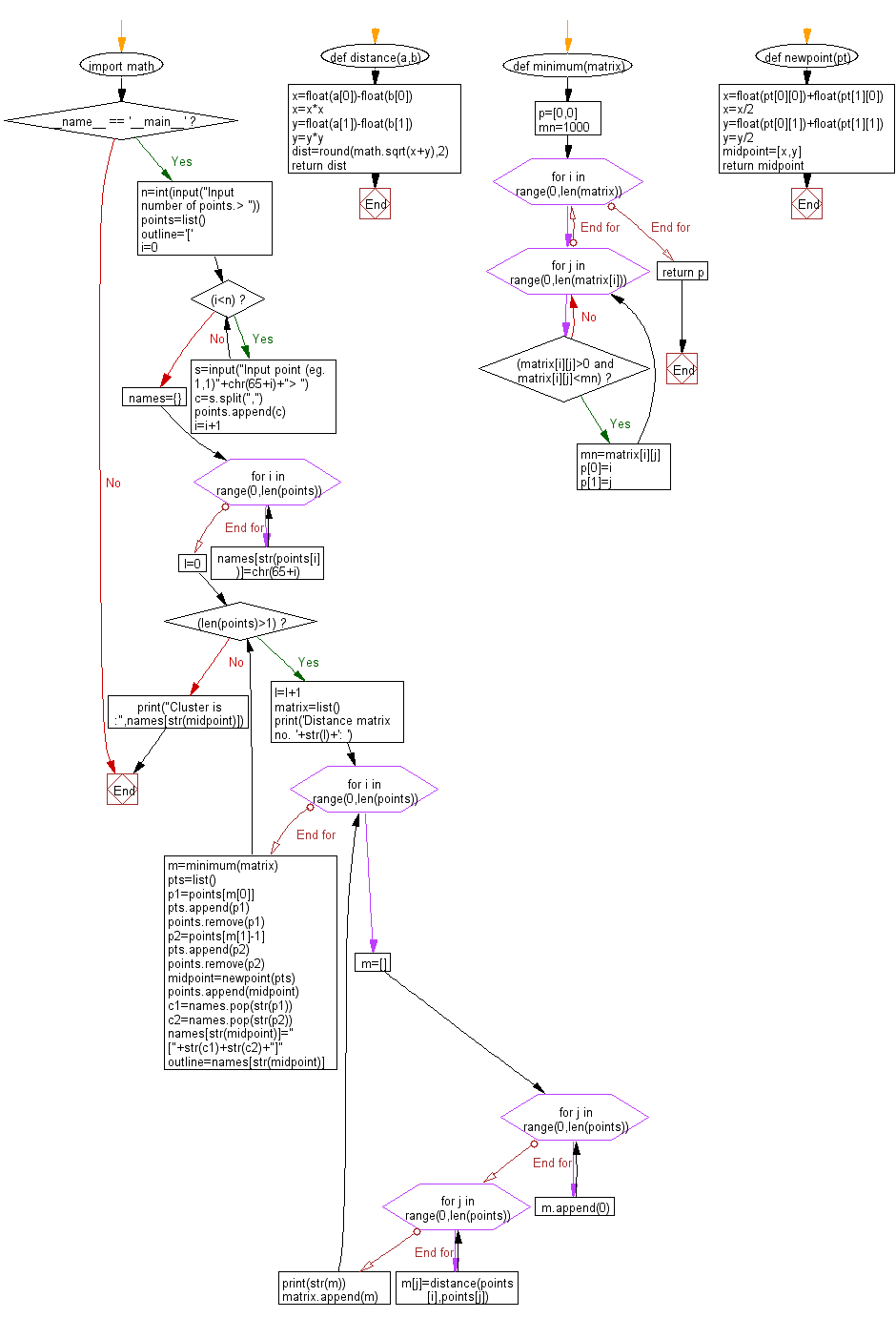﻿ Python Math: Calculate clusters using Hierarchical Clustering method - w3resource# Python Math: Calculate clusters using Hierarchical Clustering method

## Python Math: Exercise-75 with Solution

Write a Python program to calculate clusters using Hierarchical Clustering method.

Sample Solution:-

Python Code:

``````#https://gist.github.com/vineetrok/1391954

import math

def distance(a,b):
x=float(a)-float(b)
x=x*x
y=float(a)-float(b)
y=y*y
dist=round(math.sqrt(x+y),2)
return dist

def minimum(matrix):
p=[0,0]
mn=1000
for i in range(0,len(matrix)):
for j in range(0,len(matrix[i])):
if (matrix[i][j]>0 and matrix[i][j]<mn):
mn=matrix[i][j]
p=i
p=j
return p

def newpoint(pt):
x=float(pt)+float(pt)
x=x/2
y=float(pt)+float(pt)
y=y/2
midpoint=[x,y]
return midpoint

if __name__ == '__main__':
n=int(input("Input number of points.> "))
points=list()
outline='['
i=0

while(i<n):
s=input("Input point (eg. 1,1)"+chr(65+i)+"> ")
c=s.split(",")
points.append(c)
i=i+1

names={}

for i in range(0,len(points)):
names[str(points[i])]=chr(65+i)
l=0
while(len(points)>1):
l=l+1
matrix=list()
print('Distance matrix no. '+str(l)+': ')
for i in range(0,len(points)):
m=[]
for j in range(0,len(points)):
m.append(0)
for j in range(0,len(points)):
m[j]=distance(points[i],points[j])
print(str(m))
matrix.append(m)

m=minimum(matrix)
pts=list()
p1=points[m]
pts.append(p1)
points.remove(p1)
p2=points[m-1]
pts.append(p2)
points.remove(p2)
midpoint=newpoint(pts)
points.append(midpoint)
c1=names.pop(str(p1))
c2=names.pop(str(p2))
names[str(midpoint)]="["+str(c1)+str(c2)+"]"
outline=names[str(midpoint)]

print("Cluster is :",names[str(midpoint)])
```
```

Sample Output:

```Input number of points.> 2
Input point (eg. 1,1)A> 1,2
Input point (eg. 1,1)B> 3,4
Distance matrix no.1:
[0.0, 2.83]
[2.83, 0.0]
Cluster is : [AB]
```

Flowchart:## Visualize Python code execution:

The following tool visualize what the computer is doing step-by-step as it executes the said program:

Python Code Editor:

Have another way to solve this solution? Contribute your code (and comments) through Disqus.

What is the difficulty level of this exercise?

Test your Python skills with w3resource's quiz

﻿

## Python: Tips of the Day

What does if __name__ == "__main__": do?

Whenever the Python interpreter reads a source file, it does two things:

• it sets a few special variables like __name__, and then
• it executes all of the code found in the file.

Let's see how this works and how it relates to your question about the __name__ checks we always see in Python scripts.

Code Sample

Let's use a slightly different code sample to explore how imports and scripts work. Suppose the following is in a file called foo.py.

Example:

```# Suppose this is foo.py.

print("before import")
import math

print("before functionA")
def functionA():
print("Function A")

print("before functionB")
def functionB():
print("Function B {}".format(math.sqrt(100)))

print("before __name__ guard")
if __name__ == '__main__':
functionA()
functionB()
print("after __name__ guard")
```

Special Variables

When the Python interpeter reads a source file, it first defines a few special variables. In this case, we care about the __name__ variable.

When Your Module Is the Main Program

If you are running your module (the source file) as the main program, e.g.

Example:

```python foo.py
```

the interpreter will assign the hard-coded string "__main__" to the __name__ variable, i.e.

```# It's as if the interpreter inserts this at the top
# of your module when run as the main program.
__name__ = "__main__"
```

When Your Module Is Imported By Another

On the other hand, suppose some other module is the main program and it imports your module. This means there's a statement like this in the main program, or in some other module the main program imports:

```# Suppose this is in some other main program.
import foo
```

The interpreter will search for your foo.py file (along with searching for a few other variants), and prior to executing that module, it will assign the name "foo" from the import statement to the __name__ variable, i.e.

```# It's as if the interpreter inserts this at the top
# of your module when it's imported from another module.
__name__ = "foo"
```

Executing the Module's Code

After the special variables are set up, the interpreter executes all the code in the module, one statement at a time. You may want to open another window on the side with the code sample so you can follow along with this explanation.

Always

1. It prints the string "before import" (without quotes).
2. It loads the math module and assigns it to a variable called math. This is equivalent to replacing import math with the following (note that __import__ is a low-level function in Python that takes a string and triggers the actual import):
3. ```# Find and load a module given its string name, "math",
# then assign it to a local variable called math.
math = __import__("math")
```
4. It prints the string "before functionA".
5. It executes the def block, creating a function object, then assigning that function object to a variable called functionA.
6. It prints the string "before functionB".
7. It executes the second def block, creating another function object, then assigning it to a variable called functionB.
8. It prints the string "before __name__ guard".
9. Only When Your Module Is the Main Program

10. If your module is the main program, then it will see that __name__ was indeed set to "__main__" and it calls the two functions, printing the strings "Function A" and "Function B 10.0".
11. Only When Your Module Is Imported by Another

12. (instead) If your module is not the main program but was imported by another one, then __name__ will be "foo", not "__main__", and it'll skip the body of the if statement.
13. Always

14. It will print the string "after __name__ guard" in both situations.

Summary

In summary, here's what'd be printed in the two cases:

```# What gets printed if foo is the main program
before import
before functionA
before functionB
before __name__ guard
Function A
Function B 10.0
after __name__ guard
```
```# What gets printed if foo is imported as a regular module
before import
before functionA
before functionB
before __name__ guard
after __name__ guard
```

Ref: https://bit.ly/2YeDXKc